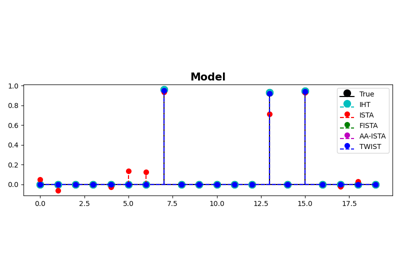# pyproximal.optimization.primal.TwIST#

pyproximal.optimization.primal.TwIST(proxg, A, b, x0, alpha=None, beta=None, eigs=None, niter=10, callback=None, show=False, returncost=False)[source]#

Two-step Iterative Shrinkage/Threshold

Solves the following minimization problem using Two-step Iterative Shrinkage/Threshold:

$\mathbf{x} = \argmin_\mathbf{x} \frac{1}{2} ||\mathbf{b} - \mathbf{Ax}||_2^2 + g(\mathbf{x})$

where $$\mathbf{A}$$ is a linear operator and $$g(\mathbf{x})$$ is any convex function that has a known proximal operator.

Parameters
proxgpyproximal.ProxOperator

Proximal operator of g function

Apylops.LinearOperator

Linear operator

bnumpy.ndarray

Data

x0numpy.ndarray

Initial vector

alphafloat, optional

Positive scalar weight (if None, estimated based on the eigenvalues of $$\mathbf{A}$$, see Notes for details)

betafloat, optional

Positive scalar weight (if None, estimated based on the eigenvalues of $$\mathbf{A}$$, see Notes for details)

eigstuple, optional

Largest and smallest eigenvalues of $$\mathbf{A}^H \mathbf{A}$$. If passed, computes alpha and beta based on them.

niterint, optional

Number of iterations of iterative scheme

callbackcallable, optional

Function with signature (callback(x)) to call after each iteration where x is the current model vector

showbool, optional

Display iterations log

returncostbool, optional

Return cost function

Returns
xnumpy.ndarray

Inverted model

jnumpy.ndarray

Cost function

Notes

The TwIST algorithm can be expressed by the following recursion:

$\mathbf{x}^{k+1} = (1-\alpha) \mathbf{x}^{k-1} + (\alpha-\beta) \mathbf{x}^k + \beta \prox_{g} (\mathbf{x}^k + \mathbf{A}^H (\mathbf{b} - \mathbf{A}\mathbf{x}^k)).$

where $$\mathbf{x}^{1} = \prox_{g} (\mathbf{x}^0 + \mathbf{A}^T (\mathbf{b} - \mathbf{A}\mathbf{x}^0))$$.

The optimal weighting parameters $$\alpha$$ and $$\beta$$ are linked to the smallest and largest eigenvalues of $$\mathbf{A}^H\mathbf{A}$$ as follows:

$\begin{split}\alpha = 1 + \rho^2 \\ \beta =\frac{2 \alpha}{\Lambda_{max} + \lambda_{min}}\end{split}$

where $$\rho=\frac{1-\sqrt{k}}{1+\sqrt{k}}$$ with $$k=\frac{\lambda_{min}}{\Lambda_{max}}$$ and $$\Lambda_{max}=max(1, \lambda_{max})$$.

Experimentally, it has been observed that TwIST is robust to the choice of such parameters. Finally, note that in the case of $$\alpha=1$$ and $$\beta=1$$, TwIST is identical to IST.

## Examples using pyproximal.optimization.primal.TwIST#ISTA, FISTA, and TWIST for Compressive sensing

ISTA, FISTA, and TWIST for Compressive sensing